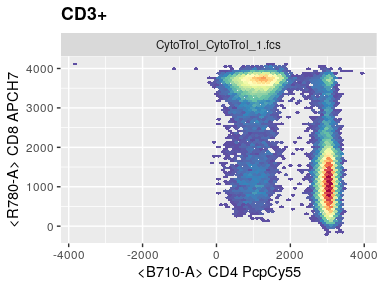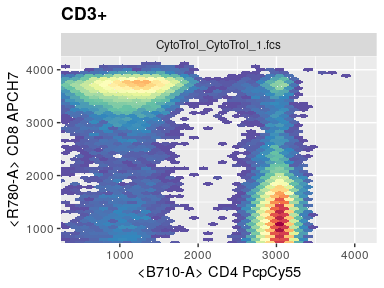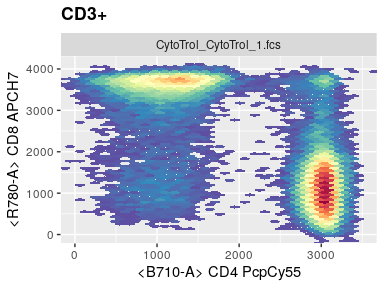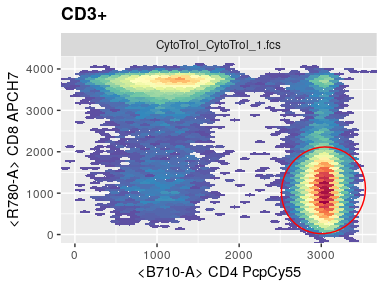By specifying the dimensions through `aes` and selecting the cell population through `subset`, `ggcyto` can easily visualize the gated data stored in `GatingSet`.

``````p <- ggcyto(gs, aes(x = CD4, y = CD8), subset = "CD3+")
# 2d plot
p <- p + geom_hex(bins = 64)
p``````## ggcyto_par_set

We can use the instrument range to automatically filter out these outlier cell events

``p + ggcyto_par_set(limits = "instrument")``Or by setting limits manually

``````myPars <- ggcyto_par_set(limits = list(x = c(0,3.5e3), y = c(-10, 4.1e3)))
p <- p + myPars# or xlim(0,3.5e3) + ylim(-10, 4e3)
p``````To check what kind of visualization parameters can be changed through `ggcyto_par_set`, simply print the default settings

``ggcyto_par_default()``
``````## \$limits
##  "data"
##
## \$facet
## <ggproto object: Class FacetWrap, Facet, gg>
##     compute_layout: function
##     draw_back: function
##     draw_front: function
##     draw_labels: function
##     draw_panels: function
##     finish_data: function
##     init_scales: function
##     map_data: function
##     params: list
##     setup_data: function
##     setup_params: function
##     shrink: TRUE
##     train_scales: function
##     vars: function
##     super:  <ggproto object: Class FacetWrap, Facet, gg>
##
## \$hex_fill
## <ScaleContinuous>
##  Range:
##  Limits:    0 --    1
##
## \$lab
## \$labels
##  "both"
##
## attr(,"class")
##  "labs_cyto"
##
## attr(,"class")
##  "ggcyto_par"``````

## geom_gate

To plot a gate, simply pass the gate name to the `geom_gate` layer

``p + geom_gate("CD4")``More than one gate can be added as long as they share the same parent and dimensions

``````p <- p + geom_gate(c("CD4","CD8")) # short for geom_gate("CD8") + geom_gate("CD4")
p``````